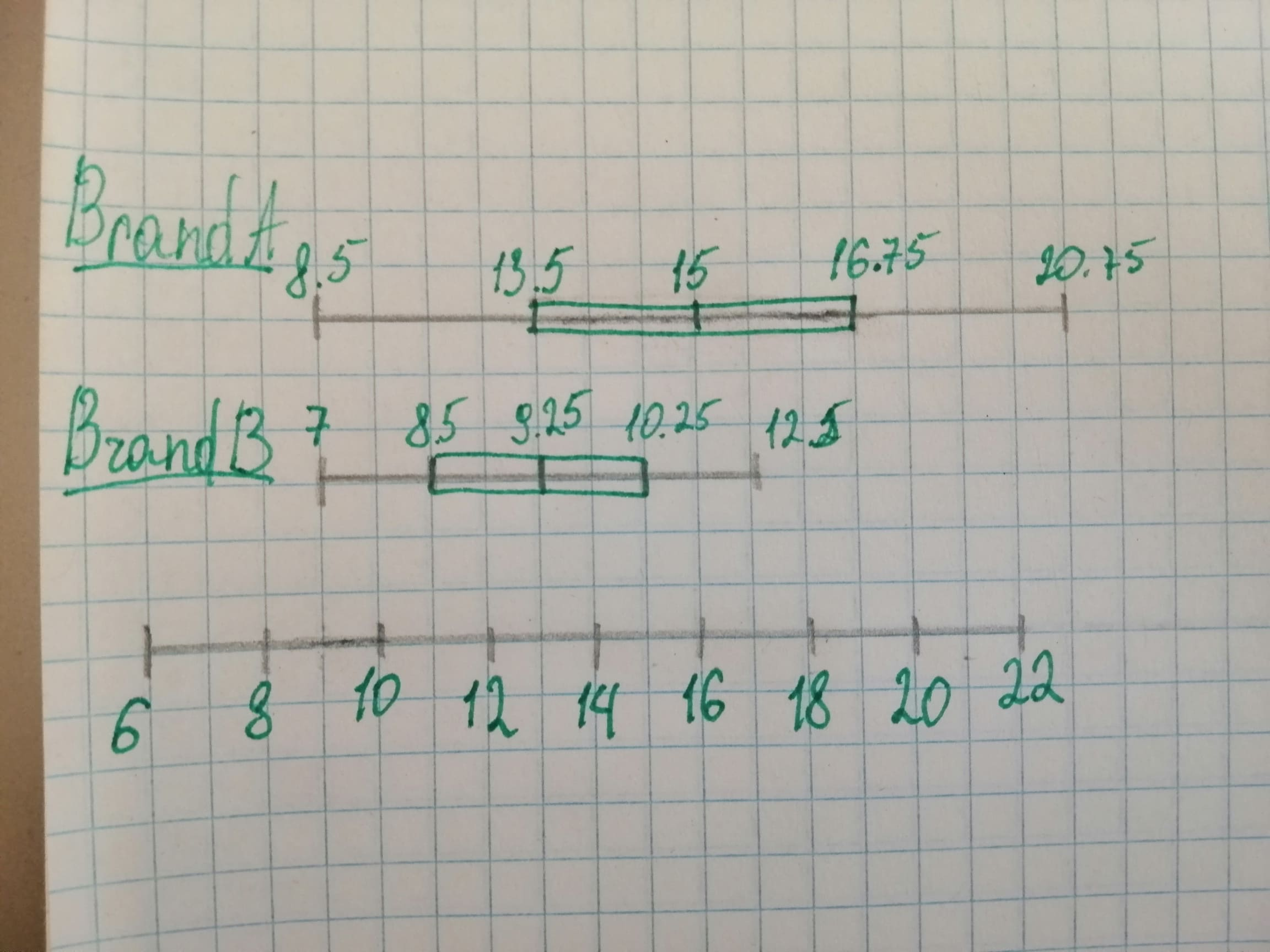# The tables show the battery lives (in hours) of two brands of laptops.a) Make a double box-and-whisker plot that represent's the data.shadsiei 2020-12-30 Answered
The tables show the battery lives (in hours) of two brands of laptops. a) Make a double box-and-whisker plot that represents
You can still ask an expert for help

• Questions are typically answered in as fast as 30 minutes

Solve your problem for the price of one coffee

• Math expert for every subject
• Pay only if we can solve itgwibdaithq

a) Double box-and-whisker plot: For brand A, Arranging the data in ascending order,
$\text{Lowest value}=8.5$
$\text{Highest value}=20.75$
$\text{Lower quartile}=13.5$
$\text{Upper quartile}=16.75$

$=15$ For brand B, Arranging the data in ascending order,
$\text{Lowest value}=7.8$
$\text{Highest value}=12.5$
$\text{Lower quartile}=8.5$
$\text{Upper quartile}=10.25$

$=9.25$b) For Brand A. The median is placed towards the left-side of the box, this means that most of the data lies on left side and distribution is skewed right. For Brand B. The median is placed towards the left-side of the box, this means that most of the data lies on left-side and distribution is skewed right. c) Brand A's battery lives are more spread out because its range is greater as compared to brand B's battery lives range d) As both distributions are skewed, median should be used as a measure of center and five-number summary for measuring variation. For brand A, median is 15 while for brand B median is 9.25.